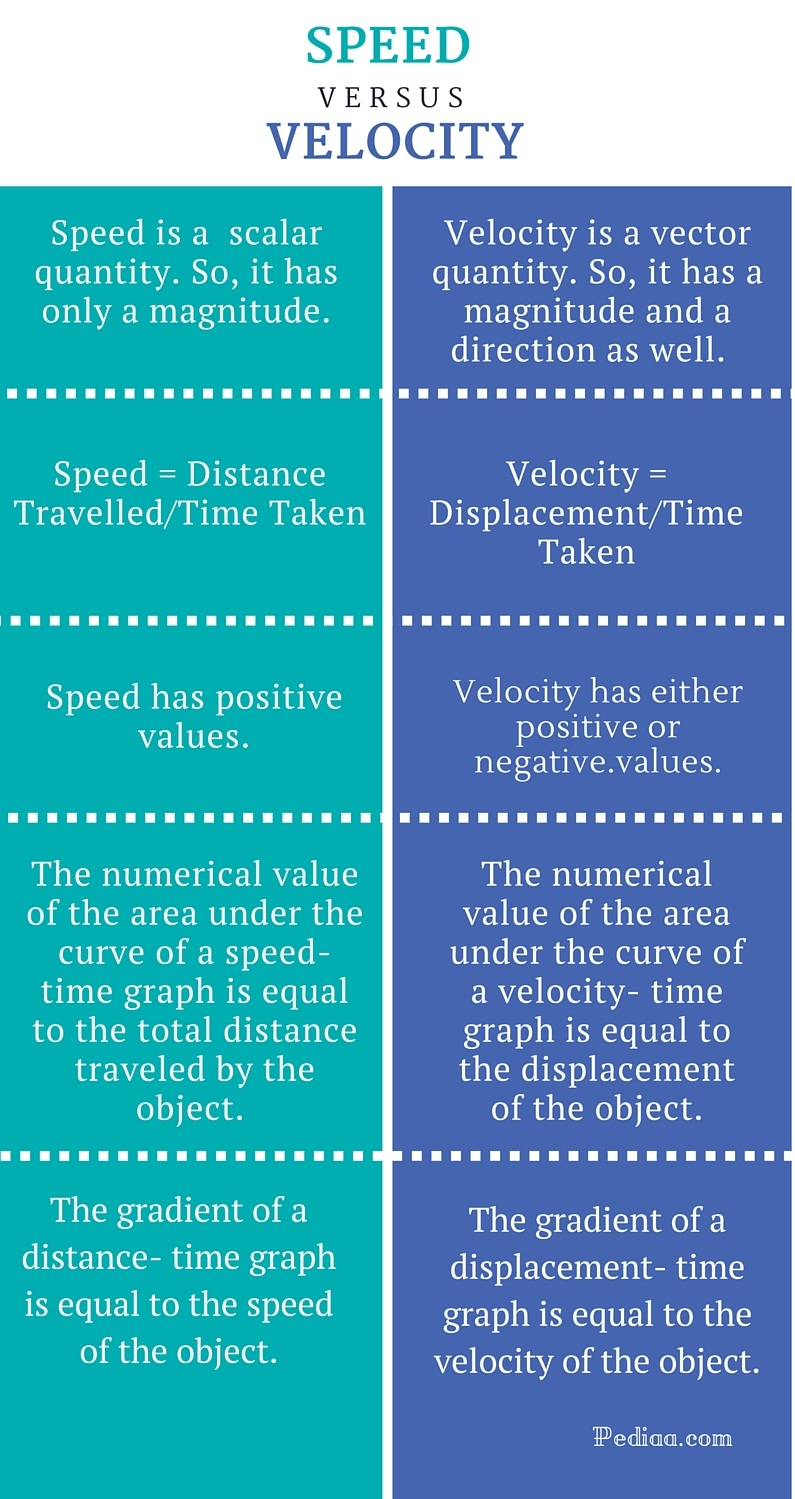# Difference Between Speed and Velocity

## Main Difference – Speed vs. Velocity

In daily life, most of the people use “speed” and “velocity” interchangeably. But in science, these two terms have different and specific meanings. Although both speed and velocity give some measurements associated with the motion of an object, velocity gives a more detailed picture of the motion compared to speed. The main difference between speed and velocity is that speed doesn’t have a directional component whereas velocity does. Therefore, in physics, velocity is a very important and frequently used quantity.

## What is Speed

Speed is the rate of change of distance of a moving body. It doesn’t contain a directional component, as it is a scalar quantity. Speed is simply a numerical value that comes with a unit. The SI unit of measuring speed is ms-1. If the distance traveled by an object and the time taken for its motion are known, its speed can be calculated using the following equation.

Speed = Distance Travelled/ Time Taken

According to the definition of the speed, any type of motion namely linear motions, rotational motions, simple harmonic motions or any combination of them has a speed. In addition, both linear and rotational motions can maintain a constant speed. But the speed of a simple harmonic motion must change at every point of time according to the definition of simple harmonic motions.## What is Velocity

Velocity is the rate of change of the displacement of a moving body. It is a vector quantity. Therefore, the velocity of an object describes both the magnitude and direction associated with its motion. The SI unit of measuring velocity is ms-1. When the velocity of an object is described, it is essential to mention both the magnitude and direction as velocity is a vector quantity. If the displacement and time taken are known, the velocity of the object can be calculated using the following equation.

Velocity = Displacement\ Time Taken

If the velocity of a body remains unchanged, the body is said to be moving with a constant velocity. If the velocity of a body is increasing with time, the body is said to be accelerating. But, if the velocity of a body is decreasing with time, the body is said to be decelerating.

According to the definition of the velocity, a body moving in a linear path, can maintain a constant velocity. But, a body moving in a rotational, simple harmonic or any combination of them cannot maintain a constant velocity. Anyhow, the magnitude of the velocity of a body moving in a circular path can remain unchanged. If the velocity of a body is changing, the body is accelerating or decelerating. So, in a circular motion, a body is always accelerating.

The velocity of a body moving in a rectilinear path with a constant speed is constant as both magnitude and direction components of the velocity aren’t changing with time. But the velocity of a body moving in a circular path with a constant speed is changing.

If the velocity of a body is changing with time (accelerating or decelerating bodies), it suits better to replace “velocity” with “instantaneous velocity”. Instantaneous velocity is the velocity of a body at any given particular instant. It is simply the derivative of the displacement with respect to time at any given particular instant. So, instantaneous velocity can be mathematically expressed as vds/dt).## Difference Between Speed and Velocity

### Nature of the Quantity:

Speed: Speed is a  scalar quantity. So, it has only a magnitude.

Velocity: Velocity is a vector quantity. So, it has a magnitude and a direction as well.

### Formula:

Speed: Speed = Distance Travelled/Time Taken

Velocity: Velocity = Displacement/Time Taken

### Sign:

Speed: Speed has positive values.

Velocity: Velocity has either positive or negative values.

Speed: ms-1

Velocity: ms-1

### Other Units:

Speed: kmh-1, mph

Velocity: kmh-1, mph

### Other Properties:

Speed: The numerical value of the area under the curve of a speed- time graph is equal to the total distance traveled by the object. The gradient of a distance- time graph is equal to the speed of the object.

Velocity: The numerical value of the area under the curve of a velocity- time graph is equal to the displacement of the object. The gradient of a displacement- time graph is equal to the velocity of the object.Image Courtesy:

“Race car- ID 080928-N-1226D-403” by U.S. Navy photo by Seaman Mass Communication Specialist Omar A. Dominquez -(Public Domain) via Commons Wikimedia

“Image 2” by U.S. Navy photo by Chief Journalist Erik Schneider. – This Image was released by the United States Navy with the ID 040501-N-1336S-037 (next). (Public Domain) via Commons Wikimedia